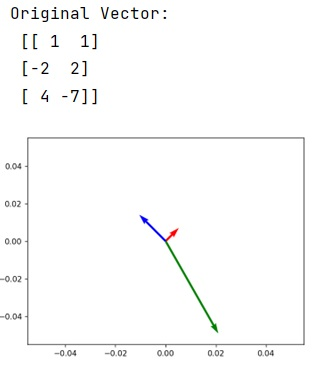# How to plot vectors using matplotlib?

Learn, how to plot vectors in Python using matplotlib?
Submitted by Pranit Sharma, on February 22, 2023

NumPy is an abbreviated form of Numerical Python. It is used for different types of scientific operations in python. Numpy is a vast library in python which is used for almost every kind of scientific or mathematical operation. It is itself an array which is a collection of various methods and functions for processing the arrays.

## Plot vectors using matplotlib

Matplotlib is a library in python programming language for creating solid visuals and interactive animations for the sake of better analytics of data.

Suppose that we are given a numpy vector/array and we need to plot this vector using matplotlib.

For this purpose, we first need to import matplotlib pyplot module with the help of which we can simply create a plot of the vector using some origin points.

Let us understand with the help of an example,

## Python code to plot vectors using matplotlib

```# Importing numpy
import numpy as np

# Importing matplotlib pyplot
import matplotlib.pyplot as plt

# Creating a vector
vec = np.array([[1,1], [-2,2], [4,-7]])

# Display original vector
print("Original Vector:\n",vec)

# Defining origin points
origin = np.array([[0, 0, 0],[0, 0, 0]])

# Creating a plot
plt.quiver(*origin, vec[:,0], vec[:,1], color=['r','b','g'], scale=21)

# Display plot
plt.show()
```

Output: